Anúncio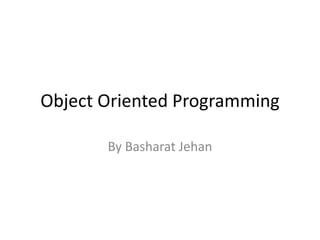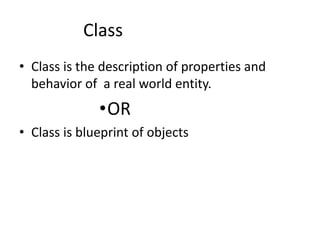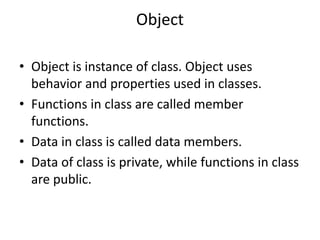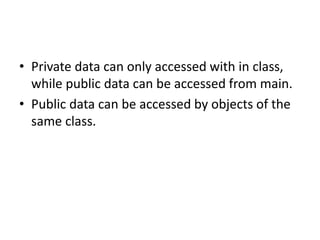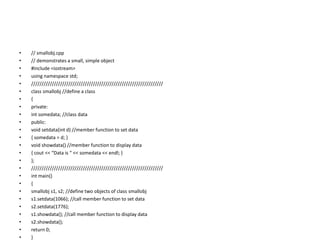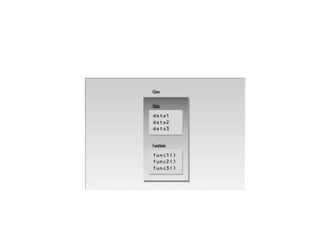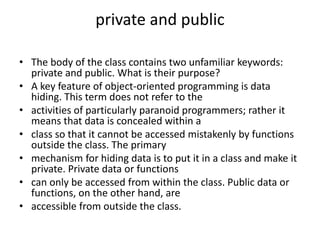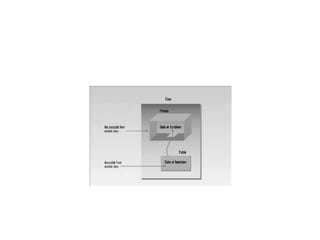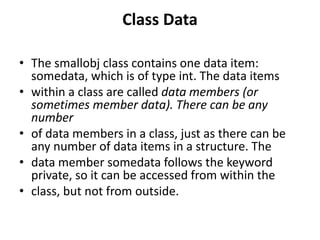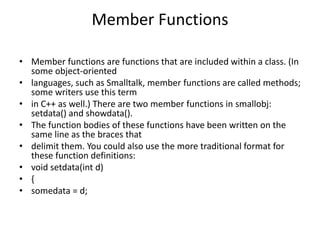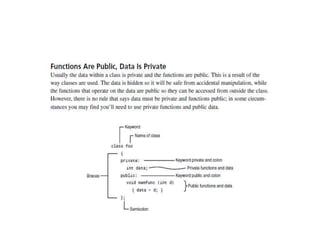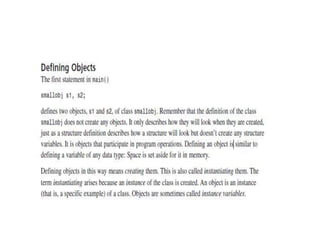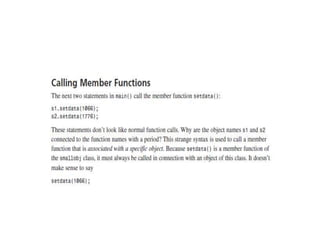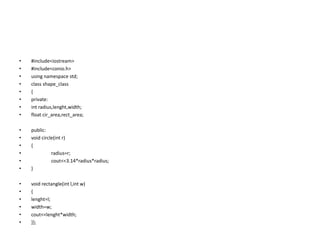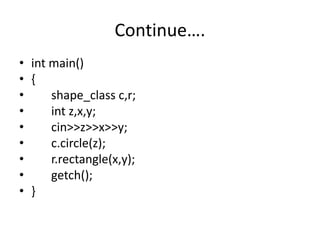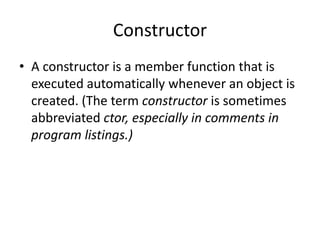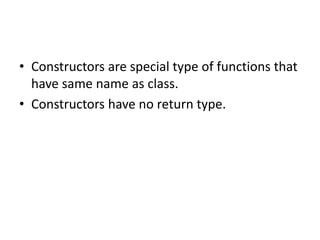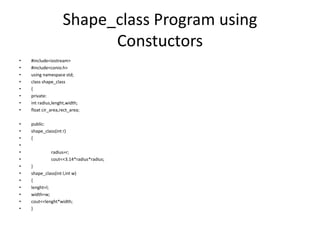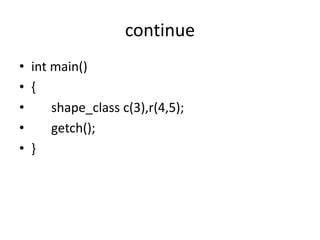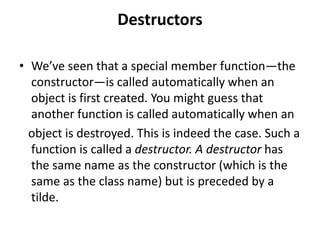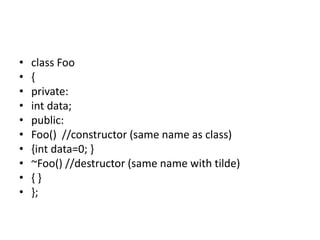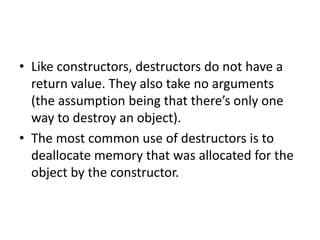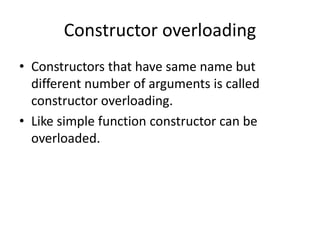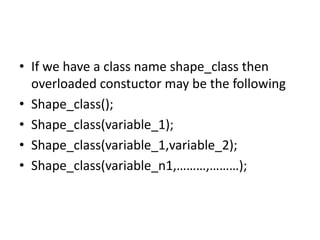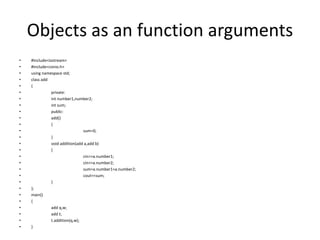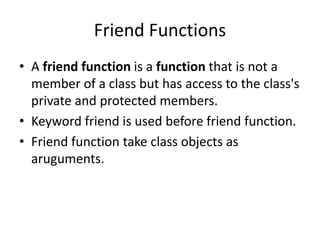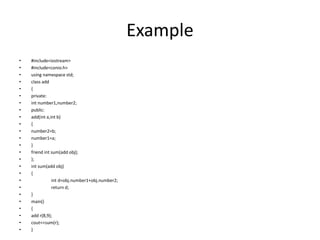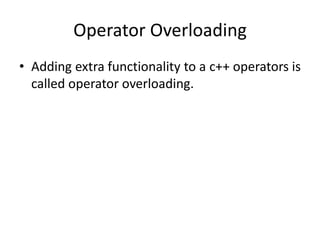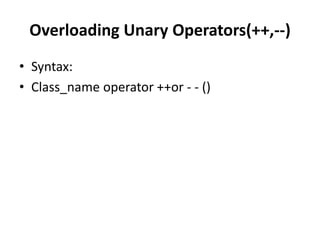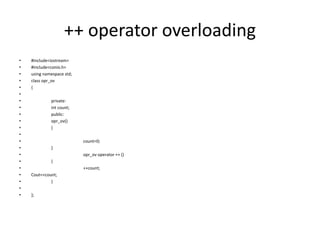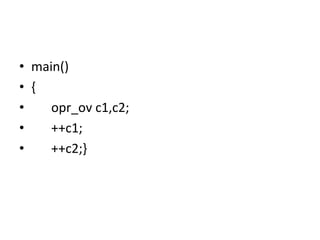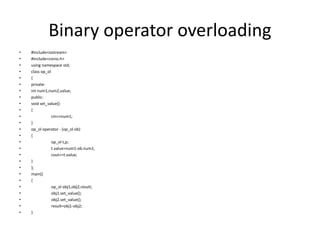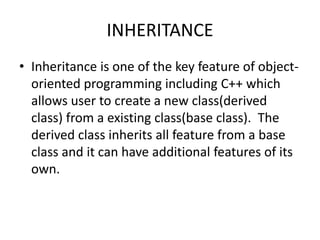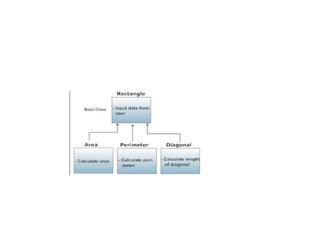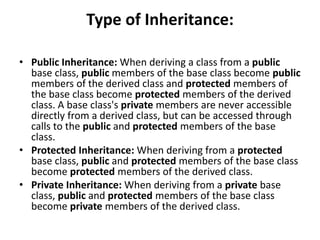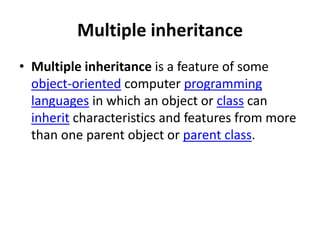1 de 37
Anúncio

### Object oriented programming in C++

1. Object Oriented Programming By Basharat Jehan
2. Class • Class is the description of properties and behavior of a real world entity. •OR • Class is blueprint of objects
3. Object • Object is instance of class. Object uses behavior and properties used in classes. • Functions in class are called member functions. • Data in class is called data members. • Data of class is private, while functions in class are public.
4. • Private data can only accessed with in class, while public data can be accessed from main. • Public data can be accessed by objects of the same class.
5. • // smallobj.cpp • // demonstrates a small, simple object • #include <iostream> • using namespace std; • //////////////////////////////////////////////////////////////// • class smallobj //define a class • { • private: • int somedata; //class data • public: • void setdata(int d) //member function to set data • { somedata = d; } • void showdata() //member function to display data • { cout << “Data is “ << somedata << endl; } • }; • //////////////////////////////////////////////////////////////// • int main() • { • smallobj s1, s2; //define two objects of class smallobj • s1.setdata(1066); //call member function to set data • s2.setdata(1776); • s1.showdata(); //call member function to display data • s2.showdata(); • return 0; • }
6. private and public • The body of the class contains two unfamiliar keywords: private and public. What is their purpose? • A key feature of object-oriented programming is data hiding. This term does not refer to the • activities of particularly paranoid programmers; rather it means that data is concealed within a • class so that it cannot be accessed mistakenly by functions outside the class. The primary • mechanism for hiding data is to put it in a class and make it private. Private data or functions • can only be accessed from within the class. Public data or functions, on the other hand, are • accessible from outside the class.
7. Hidden from Whom? • Hidden from Whom? • Don’t confuse data hiding with the security techniques used to protect computer databases. To • provide a security measure you might, for example, require a user to supply a password before • granting access to a database. The password is meant to keep unauthorized or malevolent users • from altering (or often even reading) the data. • Data hiding, on the other hand, means hiding data from parts of the program that don’t need to • access it. More specifically, one class’s data is hidden from other classes. Data hiding is designed • to protect well-intentioned programmers from honest mistakes. Programmers who really want to • can figure out a way to access private data, but they will find it hard to do so by accident.
8. Class Data • The smallobj class contains one data item: somedata, which is of type int. The data items • within a class are called data members (or sometimes member data). There can be any number • of data members in a class, just as there can be any number of data items in a structure. The • data member somedata follows the keyword private, so it can be accessed from within the • class, but not from outside.
9. Member Functions • Member functions are functions that are included within a class. (In some object-oriented • languages, such as Smalltalk, member functions are called methods; some writers use this term • in C++ as well.) There are two member functions in smallobj: setdata() and showdata(). • The function bodies of these functions have been written on the same line as the braces that • delimit them. You could also use the more traditional format for these function definitions: • void setdata(int d) • { • somedata = d;
10. • #include<iostream> • #include<conio.h> • using namespace std; • class shape_class • { • private: • int radius,lenght,width; • float cir_area,rect_area; • public: • void circle(int r) • { • radius=r; • cout<<3.14*radius*radius; • } • void rectangle(int l,int w) • { • lenght=l; • width=w; • cout<<lenght*width; • }};
11. Continue…. • int main() • { • shape_class c,r; • int z,x,y; • cin>>z>>x>>y; • c.circle(z); • r.rectangle(x,y); • getch(); • }
12. Constructor • A constructor is a member function that is executed automatically whenever an object is created. (The term constructor is sometimes abbreviated ctor, especially in comments in program listings.)
13. • Constructors are special type of functions that have same name as class. • Constructors have no return type.
14. Shape_class Program using Constuctors • #include<iostream> • #include<conio.h> • using namespace std; • class shape_class • { • private: • int radius,lenght,width; • float cir_area,rect_area; • public: • shape_class(int r) • { • • radius=r; • cout<<3.14*radius*radius; • } • shape_class(int l,int w) • { • lenght=l; • width=w; • cout<<lenght*width; • }
15. continue • int main() • { • shape_class c(3),r(4,5); • getch(); • }
16. Destructors • We’ve seen that a special member function—the constructor—is called automatically when an object is first created. You might guess that another function is called automatically when an object is destroyed. This is indeed the case. Such a function is called a destructor. A destructor has the same name as the constructor (which is the same as the class name) but is preceded by a tilde.
17. • class Foo • { • private: • int data; • public: • Foo() //constructor (same name as class) • {int data=0; } • ~Foo() //destructor (same name with tilde) • { } • };
18. • Like constructors, destructors do not have a return value. They also take no arguments (the assumption being that there’s only one way to destroy an object). • The most common use of destructors is to deallocate memory that was allocated for the object by the constructor.